# Algebra 1 : Real Numbers

## Example Questions

← Previous 1 3 4 5 6 7 8 9 25 26

### Example Question #2463 : Algebra 1

What is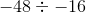?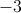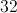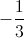Explanation:

Divide the absolute values to get the magnitude of the answer.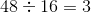Remember the following rules:

Positive divided by negative is negative.

Positive divided by positive is positive.

Negative divided by negative is positive.

Since we are dealing with two negatives, our answer will be positive.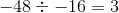### Example Question #2464 : Algebra 1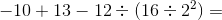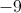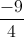Explanation:

This is a classic order of operations question, and if you are not careful, you can end up with the wrong answer!

Remember, the order of operations says that you have to go in the following order of operations: Parentheses, Exponents, Multiplication, Division, Addition, Subtraction (also known as PEMDAS).  In this equation, you will start with the parentheses.  In the parentheses, we have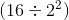.

But within the parentheses, you still need to follow PEMDAS.  First, we will solve the exponent, and the square of 2 is 4.  Then, we'll divide 16 by 4, which gives us 4, so we can rewrite our original equation as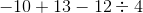.

We can now divideinto, which gives us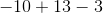The last step is to add and subtract the numbers above, paying careful attention to negative signs.  In the end, we end up withbecause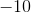added toequals, andminusequals### Example Question #2465 : Algebra 1

If a number is divisible by 9 and 12, it must also be divisible by...

I.   36

II.  48

III. 144

II only

III and II only

I and II only

III only

I only

I only

Explanation:

For a number A to be divisible by another number B, A must share all of the prime factors of B.  For example, 100 is divisible by 10 because the prime factors of 10 (5 and 2) are found in the prime factors of 100 (2, 2, 5, and 5).

In this problem, we have a number A that is divisible by 9 and 12.  First, find the prime factors of 9 and 12.  The factors of 9 are (3, 3).  The factors of 12 are (2,2,3).  So, to be divisible by 9 and 12, the number A must have the factors (2,2,3,3).  Multiply those together, and we get 2*2*3*3= 36.

So, A must be divisible by 36.  It could be divisible by the other answer choices, but since the question asked which choice must be right, we can only choose 36.

### Example Question #2466 : Algebra 1

Rewrite 100 as a number in base six.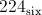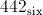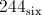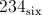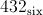Explanation:

One way to do this:

Divide 100 by 6. The remainder will be the last digit.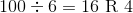Now divide the quotient by 6. This remainder will be the second-to-last digit.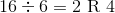The quotient is less than 6, so it is the first digit. The base-six equivalent of 100 is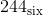.

### Example Question #2467 : Algebra 1

Divide and express the quotient in scientific notation: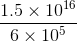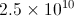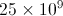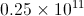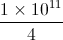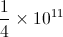Explanation:

All of these choices are equivalent to the correct answer. But onlyis in scientific notation.

### Example Question #2468 : Algebra 1

Divide: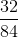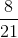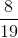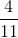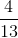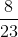Explanation:

Write the factors of the two numbers.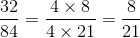### Example Question #2469 : Algebra 1

If a number is divisible by, which of the following is always true?

I. Divisible byII. Divisible byIII. Divisible byNone true

I and II

II only

All true

I only

I and II

Explanation:

If a number is divisble by some number, then it is also divisible by all of its factors. Hence, if a number is divisible by, it is also divisible by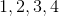, and. Thus, I and II are both true.

### Example Question #2470 : Algebra 1

Divide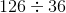.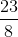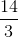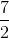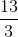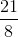Explanation:

Rewrite the expression in fraction form.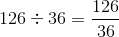Write out the common factors of both the numerator and denominator.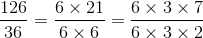We can then see that the fraction can be reduced towithout having to conduct long division.

The answer is.

### Example Question #1 : Real Numbers

Divide: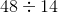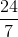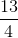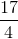Explanation:

Rewrite the expression as a fraction.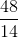Write out the multiples of the numerator and denominator.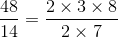The twos can be cancelled.  Simplify what remains of the fraction.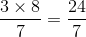The answer is:### Example Question #2 : Real Numbers

Divide the integers: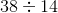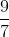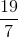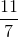Explanation:

Rewrite the expression as a fraction.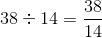Rewrite the fraction by common factors of two.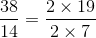Divide and cancel the twos, which will become one.

The answer is:← Previous 1 3 4 5 6 7 8 9 25 26